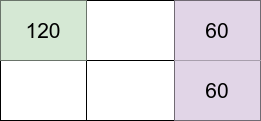# 最大化网格幸福感

## 1659. 最大化网格幸福感 (Hard)

• 内向的人 开始 时有 `120` 个幸福感，但每存在一个邻居（内向的或外向的）他都会 失去  `30` 个幸福感。
• 外向的人 开始 时有 `40` 个幸福感，每存在一个邻居（内向的或外向的）他都会 得到  `20` 个幸福感。```输入：m = 2, n = 3, introvertsCount = 1, extrovertsCount = 2

- 位于 (1,1) 的内向的人的幸福感：120（初始幸福感）- (0 * 30)（0 位邻居）= 120
- 位于 (1,3) 的外向的人的幸福感：40（初始幸福感）+ (1 * 20)（1 位邻居）= 60
- 位于 (2,3) 的外向的人的幸福感：40（初始幸福感）+ (1 * 20)（1 位邻居）= 60

```

```输入：m = 3, n = 1, introvertsCount = 2, extrovertsCount = 1

- 位于 (1,1) 的内向的人的幸福感：120（初始幸福感）- (1 * 30)（1 位邻居）= 90
- 位于 (2,1) 的外向的人的幸福感：40（初始幸福感）+ (2 * 20)（2 位邻居）= 80
- 位于 (3,1) 的内向的人的幸福感：120（初始幸福感）- (1 * 30)（1 位邻居）= 90

```

```输入：m = 2, n = 2, introvertsCount = 4, extrovertsCount = 0

```

• `1 <= m, n <= 5`
• `0 <= introvertsCount, extrovertsCount <= min(m * n, 6)`

### 相关话题

[位运算] [记忆化搜索] [动态规划] [状态压缩]